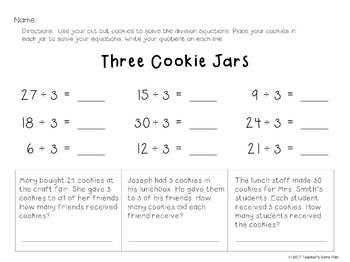3rd - 4th, Homeschool
Subjects
Standards
Resource Type
Formats Included
• Zip
Pages
34 pages

### Description

Great to use after a few days of teaching your students how division works. These task cards have division problems with matching arrays or objects, making equal groups, repeated subtraction, fact families, solving division equations, and labeling the dividend, divisor, and quotient.

Students will use the following vocabulary words: division equation, repeated subtraction, dividend, divisor, quotient, expression, array, fact family, even number, equation, and divided by.

Use these 32 Intro to Division task cards for traditional SCOOT activities, small guided math groups, enrichment or reteaching as needed. Great for math centers or to practice with a buddy in the morning.

Students use the cookies and cookie jars to learn how to share equally. Focus on the 2s divisors and use the two cookie jars as students learn the concept of sharing equally. Separate cookie jar sorting mats for the 2s through the 10s divisors. Students also have a matching division equation and word problems page to use with the cookie jar sorting mats. Great to use in a math center or guided math groups.

Thank you,
Teacher's Game Plan

Total Pages
34 pages
Included
Teaching Duration
N/A
Report this Resource to TpT
Reported resources will be reviewed by our team. Report this resource to let us know if this resource violates TpT’s content guidelines.

### Standards

to see state-specific standards (only available in the US).
Understand division as an unknown-factor problem. For example, find 32 ÷ 8 by finding the number that makes 32 when multiplied by 8.
Determine the unknown whole number in a multiplication or division equation relating three whole numbers. For example, determine the unknown number that makes the equation true in each of the equations 8 × ? = 48, 5 = __ ÷ 3, 6 × 6 = ?.
Use multiplication and division within 100 to solve word problems in situations involving equal groups, arrays, and measurement quantities, e.g., by using drawings and equations with a symbol for the unknown number to represent the problem.
Interpret whole-number quotients of whole numbers, e.g., interpret 56 ÷ 8 as the number of objects in each share when 56 objects are partitioned equally into 8 shares, or as a number of shares when 56 objects are partitioned into equal shares of 8 objects each. For example, describe a context in which a number of shares or a number of groups can be expressed as 56 ÷ 8.